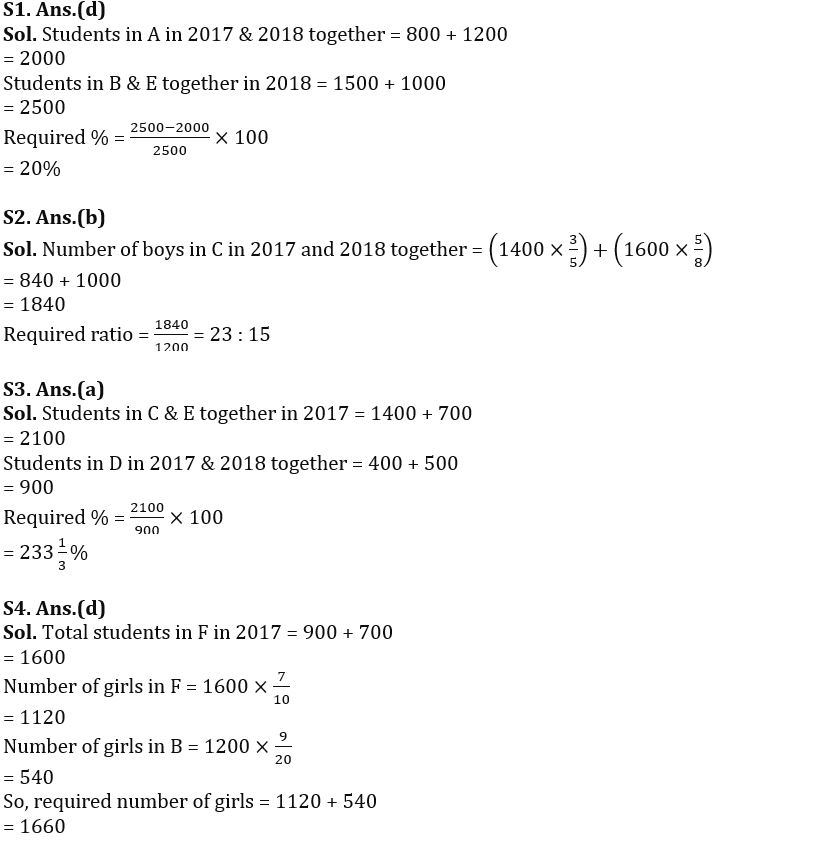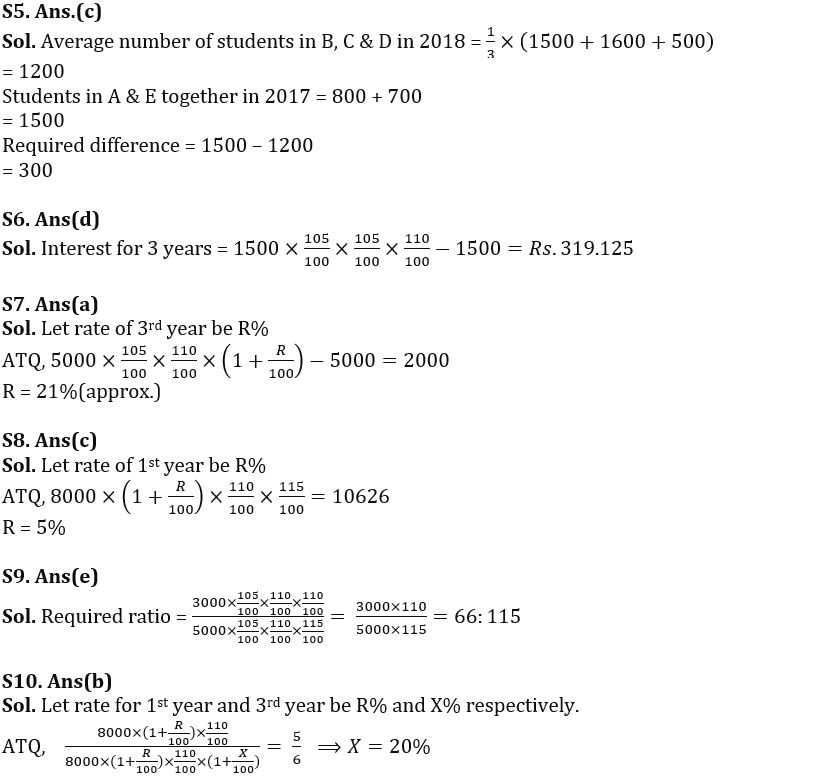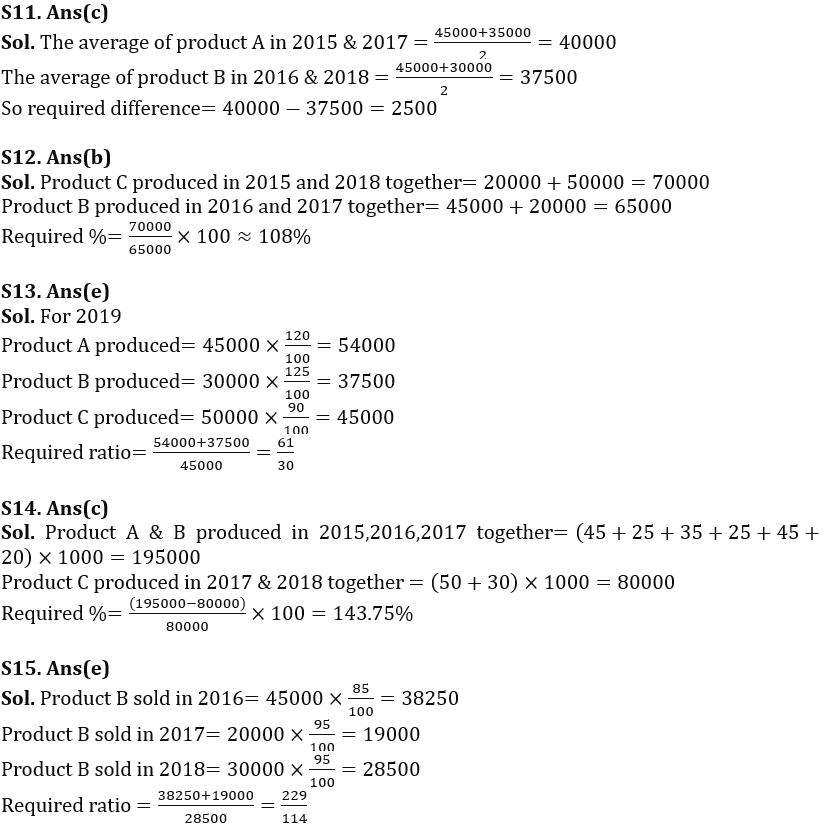SBI

# Quantitative Aptitude Quiz for SBI PO Recruitment 2022- 1st December | Rare Techy

The radar map shows the number of students (in ’00) in 5 different schools (A, B, C, D & E) in 2017 & 2018.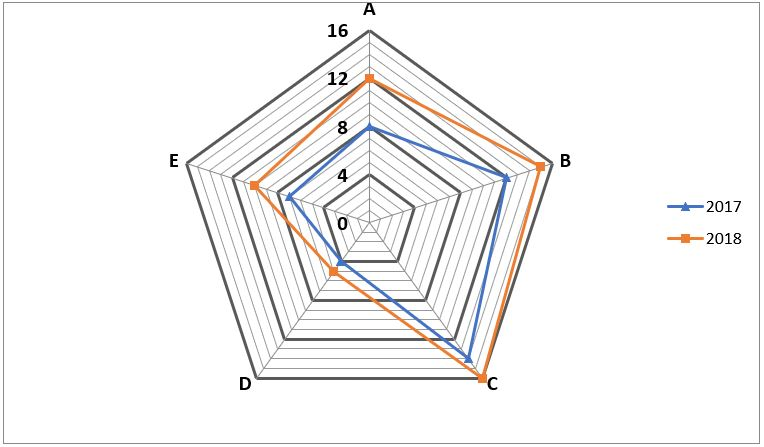Q1. What percentage of students in A combined in 2017 and 2018 are more or less than B & E students combined in 2018?
(a) 80%
(b) 30%
(c) 50%
(d) 20%
(e) 60%

Q2. If the ratio of boys and girls in C in 2017 and 2018 is 3:2 and 5:3 respectively, then find the ratio of the number of boys in C in 2017 and 2018 to the total number of students in B in 2017.
(a) 7:5
(b) 23:15
(c) 3:2
(d) 21:13
(e) 4:3

Q3. Students in C & E combined in 2017 are what percent of students in D combined in 2017 and 2018?
(a) 233⅓%
(b) 266⅔%
(c) 250%
(d) 225%
(e) 275%

Q4. If the total number of students in F in 2017 is 900 more than the total number of students in E in 2017 and the ratio of boys and girls in B & F in 2017 is 11 respectively: 9 and 3: 7 then find the number of girls in B & F together. 2017
(a) 1450
(b) 1280
(c) 1340
(d) 1660
(e) None of the above.

Q5. Is the average number of students in B, C & D in 2018 more or less than the number of students in A & E combined in 2017?
(a) 100
(b) 200
(c) 300
(d) 500
(e) 400

Exercises (6-10):- The given table provides the rate of interest on a given amount in year 1, 2, 3 and 4. All annual calculations should be done according to the compound interest accrual. There is some missing data that you should find using the information provided in the question.
Study the data carefully and answer the questions.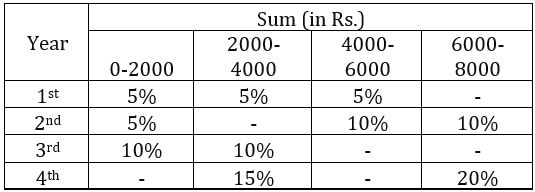[NOTE:- interest rate is given year wise not for the period.]

Q6. What is the interest on the amount of Rs. 1500 for 3 years?
(a) Rs. 300
(b) Rs. 180
(c) Rs. 375
(d) Rs. 319.125
(e) Rs. 325

Q7. If the interest on the amount of Rs. 5000 in 3 years. 2000. Find the interest rate for the 3rd year. (close to)
(a) 21%
(b) 23%
(c) 19%
(d) 25%
(e) 27%

Q8. Akshay invested Rs. 8000 offers an interest in a scheme mentioned in the table. After 3 years it is Rs. 10626. If the scheme provides the third year interest rate as 15% pa, then find the 1st year rate.
(a) 3%
(b) 4%
(c) 5%
(d) 6%
(e) 10%

Q9. What is the percentage of the amount received on a sum of Rs. 3000 for 3 years (if the 2nd year interest rate is equal to the 3rd year interest rate) for an amount received on a sum of Rs. 5000 for 3 years (if the interest rate in year 3 is 50% more than that in year 2)?
(a) 33:115
(b) 22:115
(c) 3:5
(d) 22:23
(e) 66:115

Q10. The percentage of amount received on investment of Rs. 8000 for 2 years and 3 years is 5:6. Find the interest rate for the 3rd year.
(a) 15%
(b) 20%
(c) 25%
(d) Not specified
(e) None of these

Directions (11-15): Study the sequence chart given below and answer the following questions.
The line chart shows the quantity (in ‘000) of different products produced by company ‘X’ in different years.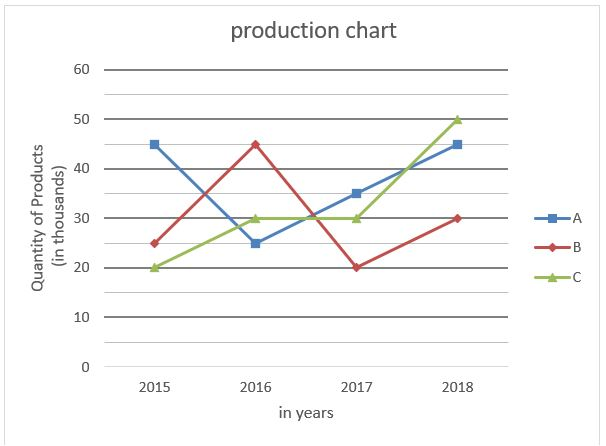Q11. Find the difference between the average of product A produced in 2015 and 2017 and the average of product B produced in 2016 and 2018?
(a) 4500
(b) 3500
(c) 2500
(d) 1500
(e) 5000

Q12. Product C produced in 2015 and 2018 combined is what percentage of product B produced in 2016 and 2017 combined?
(a) 105%
(b) 108%
(c) 110%
(d) 128%
(e) 115%

Q13. In the year 2019, if the production of product A & B shows an increase in their production of 20% and 25% respectively while the production of product C has decreased by 10% compared to the previous year, then the ratio of product A & B together see produced. in 2019 than that of product C produced in 2019.
(a) 19/30
(b) 43/30
(c) 47/30
(d) 41/30
(e) 61/30

Q14. Product A and B produced together in 2015, 2016 and 2017 are by what percentage more/less than product C produced together in 2017 and 2018?
(a) 250%
(b) 200%
(c) 143.75%
(d) 133.33%
(e) 125%

Question 15. If 15% of product B produced in 2016 remains unsold in 2017 and 95% in 2018, then the percentage of product B produced in 2016 and 2017 is or sold together with what was sold in 2018? (total production = sold + unsold)
(a) 109/114
(b) 259/114
(c) 119/109
(d) 229/109
(e) 229/114

Solutions